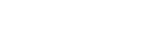# MATH221 - Calculus I

## General

MATH

221

00161721

Lecture

### Description

Limits, continuity, differentiation, applications of derivatives, integration. Prereq.: MATH 115, or MATH 112 and MATH 113, or high school advanced algebra and trigonometry with a satisfactory math placement score.

### Notes

Go to www.stcloudstate.edu/mathplacement Placement req prior to Registration

4

4

No

Yes

04

No

## Learning Outcomes

### Outcome

Calculate limits using graphical, algebraic and numerical methods and the Squeeze Theorem.

### Outcome

Determine continuity of a function at points, identify various types of discontinuities and identify intervals of continuity for functions.

### Outcome

Calculate derivatives from the definition and using differentiation rules and implicit differentiation.

### Outcome

Use differentiation to identify tangent lines, to solve geometric problems and to solve problems involving rates of change.

### Outcome

Solve optimization and related-rates problems by analytic methods.

### Outcome

Graph a function, showing all relevant information such as relative extrema, inflection points, and asymptotes, with analytic methods and without the aid of graphing technology.

### Outcome

Approximate roots of equations by using the bisection method and Newton¿s method.

### Outcome

Calculate integrals by using the Fundamental Theorem of Calculus, u-substitution, and observations about symmetry of graphs.

### Outcome

Use integration to find the area under a curve, to solve problems involving net change, and to solve other geometric problems.

### Outcome

State and apply named theorems of calculus (the Intermediate Value Theorem, the Mean Value Theorem, and the Fundamental Theorem of Calculus).

### Outcome

Identify whether a derivative or an integral (or neither) is more appropriate for use in a given problem.

### Outcome

Communicate their knowledge of the basic principles of Calculus I, both orally (e.g. class discussions) and in writing (e.g. written assessments).

-

## Dependencies

No dependencies720 4th Avenue South
St. Cloud, MN 56301-4498
(320) 308-0121St. Cloud State University
A member of Minnesota State and committed to legal affirmative action, equal opportunity, access and diversity of its campus community (Full Statement).
© 2021 St. Cloud State University